Thursday, August 5, 2021
Home > geometry > Geometry Questions for CAT | CAT Exam Geometry Questions Bank PDF

# Geometry Questions for CAT | CAT Exam Geometry Questions Bank PDF# Geometry Questions for CAT |

Hi CAT aspirants, welcome to Amans Maths Blogs (AMB). On this post, you will get the collection of CAT Exam Geometry Questions Bank PDF of previous year geometry questions of CAT exam from 1990 to 2018.

## CAT Exam Geometry Questions Bank PDF : 1990

CAT Exam Geometry Questions Bank PDF: Ques No 1

A square is drawn by joining the midpoints of the sides of a given square. A third square is drawn inside the second square in the same way and this process is continued indefinitely. If a side of the first square is 8 cm, the sum of the areas of all the squares such formed (in sq.cm.) is [CAT 1990]

Options:

A. 128

B. 120

C. 96

D. None of These

Solution:

## CAT Exam Geometry Questions Bank PDF : 1991

CAT Exam Geometry Questions Bank PDF: Ques No 2

Let the consecutive vertices of a square S be A,B,C &D. Let E,F & G be the mid-points of the sides B, BC & AD respectively of the square. Then the ratio of the area of the quadrilateral EFDG to that of the square S is nearest to [CAT 1991]

Options:

A. 1/2

B. 1/3

C. 1/4

D. 1/8

Solution:

CAT Exam Geometry Questions Bank PDF: Ques No 3

A circle is inscribed in a given square and another circle is circumscribed about the square. What is the ratio of the area of the inscribed circle to that of the circumscribed circle? [CAT 1991]

Options:

A. 2:3

B. 3:4

C. 1:4

D. 1:2

Solution:

## CAT Exam Geometry Questions Bank PDF : 1993

CAT Exam Geometry Questions Bank PDF: Ques No 4

What is the area of a regular hexagon?

I. The length of the boundary line of the hexagon is 36 cm.

II. The area of the hexagon is 6 times the area of an equilateral. [CAT 1993]

Options:

A. If the question can be answered with the help of statement I alone.

B. If the question can be answered with the help of statement II alone.

C. If both, statement I and statement II are needed to answer the question.

D. If the question cannot be answered even with the help of both the statements.

Solution:

CAT Exam Geometry Questions Bank PDF: Ques No 5

The line AB is 6 meters in length and is tangent to the inner one of the two concentric circles at point C. It is known that the radii of the two circles are integers. The radius of the outer circle is [CAT 1993]

Options:

A. 5 meter

B. 4 meter

C. 6 meter

D. 3 meter

Solution:

## Geometry Questions for CAT : 1994

CAT Exam Geometry Questions Bank PDF: Ques No 6

Four friends start from four towns, which are at the four corners of an imaginary rectangle. They meet at a point which falls inside the rectangle, after travelling distances of 40, 50 and 60 metres. The maximum distance that the fourth could have traveled is (approximately) [CAT 1994]

Options:

A. 67 meter

B. 52 meter

C. 22.5 meter

D. Cannot be Determined

Solution:

CAT Exam Geometry Questions Bank PDF: Ques No 7

In a triangle ACD, AD = AC and angle C = 2(angle E). The distance between parallel lines AB and CD is h. Then

I. Area of Parallelogram ABCD and

II. Area of triangle ADE [CAT 1994]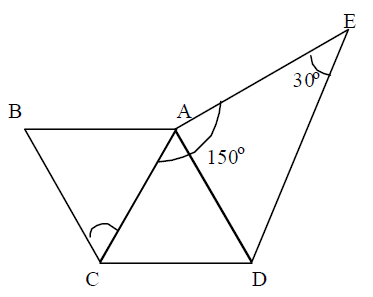Options:

A. If I > II

B. If I < II

C. If I = II

D. If nothing can be said

Solution:

CAT Exam Geometry Questions Bank PDF: Ques No 8

k1, k2, k3 are parallel lines. AD = 2 cm, BE = 8 CM and CF = 32 cm

I. (AB) x (EF)

II. (CD) x (DE) [CAT 1994]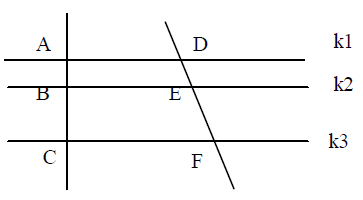Options:

A. If I > II

B. If I < II

C. If I = II

D. If nothing can be said

Solution:

CAT Exam Geometry Questions Bank PDF: Ques No 9

Is segment PQ greater than segment RS?

I. PB > RE, BQ = ES

II. B is a point on PQ, E is a point on RS [CAT 1994]

Options:

A. If the question can be answered with the help of statement I alone

B. If the question can be answered with the help of statement I, alone

C. If both, statement I and statement II are needed to answer the question, and

D. If the question cannot be answered even with the help of both the statements.

Solution:

## Geometry Questions for CAT : 1995

CAT Exam Geometry Questions Bank PDF: Ques No 10

Is ABCD is a square of area 4, which is divided into four non-over lapping triangles as shown in figure. Then the sum of the perimeters of the triangles is [CAT 1995]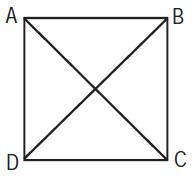Options:

A. 8(2 + √2)

B. 8(1+ √2)

C. 4(1 + √2)

D. 4(2 + √2)

Solution:

CAT Exam Geometry Questions Bank PDF: Ques No 11

AB ⊥ BC, BD ⊥ AC and CE bisects ∠C, ∠A = 30°. Then what is ∠CED [CAT 1995]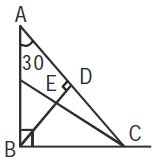Options:

A. 30 Degrees

B. 45 Degrees

C. 65 Degrees

D. 60 Degrees

Solution:

CAT Exam Geometry Questions Bank PDF: Ques No 12

PQRS is a square. SR is a tangent (at point S) to the circle with center O and TR = OS. Then the ratio of area of the circle to the area of the square is [CAT 1995]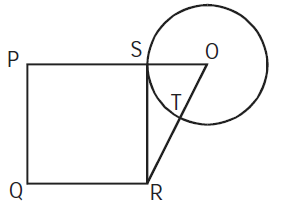Options:

A. Pi/3

B. 11/7

C. 3/Pi

D. 7/11

Solution:

CAT Exam Geometry Questions Bank PDF: Ques No 13

The sides of a triangle are 5, 12 and 13 units. A rectangle is constructed, which is equal in area to the triangle, and has a width of 10 units. Then the perimeter of the rectangle is [CAT 1995]

Options:

A. 30

B. 36

C. 13

D. None of these

Solution:

CAT Exam Geometry Questions Bank PDF: Ques No 14

In the adjoining figure, AC+ AB = 5AD and AC – AD = 8. Then the area of the rectangle ABCD is [CAT 1995]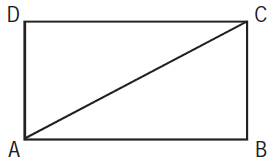Options:

A. 36

B. 50

C. 60

D. Cannot be determined

Solution:

CAT Exam Geometry Questions Bank PDF: Ques No 15

In the given figure, AB is diameter of the circle and points C and D are on the circumference such that ∠CAD = 30° and ∠CBA = 70°. What is the measure of ∠ACD? [CAT 1995]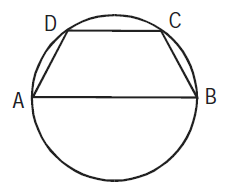Options:

A. 40 Degree

B. 30 Degree

C. 50 Degree

D. 90 Degree

Solution:

CAT Exam Geometry Questions Bank PDF: Ques No 16

The length of a ladder is exactly equal to the height of the wall it is learning against. If lower end of the ladder is kept on a stool of height 3 m and the stool is kept 9 m away from the wall, the upper end of the ladder coincides with the top of the wall. Then the height of the wall is [CAT 1995]

Options:

A. 12 m

B. 15 m

C. 18 m

D. 11 m

Solution:

CAT Exam Geometry Questions Bank PDF: Ques No 17

A, B, C and D are four towns any three of which are non-collinear. Then the number of ways to construct three roads each joining a pair of towns so that the roads do not form a triangle is [CAT 1995]

Options:

A. 7

B. 8

C. 9

D. 24

Solution:

CAT Exam Geometry Questions Bank PDF: Ques No 18

What is the area of the triangle?

I. Two sides are 41 cm each.

II. The altitude to the third side is 9 cm long. [CAT 1995]

Options:

A. If the question can be answered with the help of statement I alone

B. If the question can be answered with the help of statement II, alone

C. If both statement I and statement II are needed to answer the question.

D. If the question cannot be answered even with the help of both the statements

Solution:

CAT Exam Geometry Questions Bank PDF: Ques No 19

What is the length of rectangle ABCD?

I. Area of the rectangle is 48 square units.

II. Length of the diagonal is 10 units. [CAT 1995]

Options:

A. If the question can be answered with the help of statement I alone

B. If the question can be answered with the help of statement II, alone

C. If both statement I and statement II are needed to answer the question.

D. If the question cannot be answered even with the help of both the statements

Solution:

## Geometry Questions for CAT : 1996

CAT Exam Geometry Questions Bank PDF: Ques No 20

If ABCD is a square and BCE is an equilateral triangle, what is the measure of ∠DEC [CAT 1996]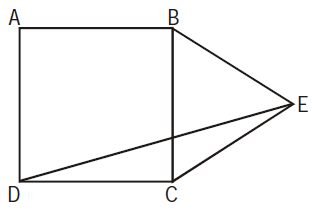Options:

A. 15 Degree

B. 20 Degree

C. 30 Degree

D. 45 Degree

Solution:

CAT Exam Geometry Questions Bank PDF: Ques No 21

From a circular sheet of paper with a radius 20 cm, four circles of radius 5 cm each are cut out. What is the ratio of the uncut to the cut portion? [CAT 1996]

Options:

A. 1:3

B. 4:1

C. 3:1

D. 4:3

Solution:

CAT Exam Geometry Questions Bank PDF: Ques No 22

The figure shows a circle of diameter AB and radius 6.5 cm. If chord CA is 5 cm long, find the area of ΔABC.  [CAT 1996]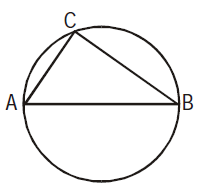Options:

A. 60 sq. unit

B. 30 sq. unit

C. 40 sq. unit

D. 52 sq. unit

Solution:

CAT Exam Geometry Questions Bank PDF: Ques No 23

In ΔABC, ∠B is a right angle, AC = 6 cm, and D is the mid-point of AC. The length of BD is [CAT 1996]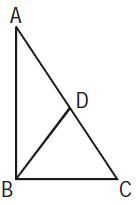Options:

A. 4 cm

B.cm

C. 3 cm

D. 3.5 cm

Solution:

CAT Exam Geometry Questions Bank PDF: Ques No 24

The figure shows the rectangle ABCD with a semicircle and a circle inscribed inside in it as shown.
What is the ratio of the area of the circle to that of the semicircle?
[CAT 1996]Options:

A. (Root(2) – 1)2 : 1

B. 2(Root(2) – 1)2 : 1

C. (Root(2) – 1)2 : 2

D. None of these

Solution:

## Geometry Questions for CAT : 1997

CAT Exam Geometry Questions Bank PDF: Ques No 25

The sum of the areas of two circles, which touch each other externally, is 153π. If the sum of their
radii is 15, find the ratio of the larger to the smaller radius
[CAT 1997]

Options:

A. 4

B. 2

C. 3

D. None of these

Solution:

CAT Exam Geometry Questions Bank PDF: Ques No 26

AB is the diameter of the given circle, while points C and D lie on the circumference as shown. If AB
is 15 cm, AC is 12 cm and BD is 9 cm, find the area of the quadrilateral ACBD
[CAT 1997]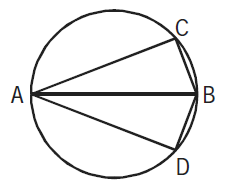Options:

A. 54Pi

B. 216Pi

C. 162Pi

D. None of these

Solution:

CAT Exam Geometry Questions Bank PDF: Ques No 27

The adjoining figure shows a set of concentric squares. If the diagonal of the innermost square is
2 units, and if the distance between the corresponding corners of any two successive squares is
1 unit, find the difference between the areas of the eighth and the seventh squares, counting from
the innermost square.
[CAT 1997]Options:

A. 10√2 sq. units

B. 30 sq. units

C. 35√2 sq. units

D. None of these

Solution:

CAT Exam Geometry Questions Bank PDF: Ques No 28

The value of each of a set of coins varies as the square of its diameter, if its thickness remains constant, and it varies as the thickness, if the diameter remains constant. If the diameter of two coins are in the ratio 4 : 3, what should be the ratio of their thickness’ be if the value of the first is four times that of the second? [CAT 1997]

Options:

A. 16 : 9

B. 9 : 4

C. 9 : 16

D. 4 : 9

Solution:

CAT Exam Geometry Questions Bank PDF: Ques No 29

In ΔABC, points P, Q and R are the mid-points of sides AB, BC and CA respectively. If area of ΔABC is 20 sq. units, find the area of ΔPQR [CAT 1997]

Options:

A. 10 sq. units

B. 5√3 sq. units

C. 5 sq. units

D. None of these

Solution:

CAT Exam Geometry Questions Bank PDF: Ques No 30

In a rectangle, the difference between the sum of the adjacent sides and the diagonal is half the length of the longer side. What is the ratio of the shorter to the longer side? [CAT 1997]

Options:

A. √3 : 2

B. 1 : √3

C. 2 : 5

D. 3 : 4

Solution:

CAT Exam Geometry Questions Bank PDF: Ques No 31

In the adjoining figure, points A, B, C and D lie on the circle. AD = 24 and BC = 12. What is the ratio of the area of Δ CBE to that of Δ ADE? [CAT 1997]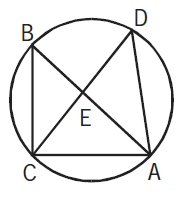Options:

A. 1 : 4

B. 1 : 2

C. 1 : 3

D. 3 : 4

Solution:

CAT Exam Geometry Questions Bank PDF: Ques No 32

In the given figure, EADF is a rectangle and ABC is a triangle whose vertices lie on the sides of EADF and AE = 22, BE = 6, CF = 16 and BF = 2. Find the length of the line joining the mid-points of the sides AB and BC. [CAT 1997]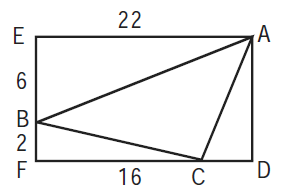Options:

A. 4Root(2)

B. 5

C. 3 : 5

D. None of these

Solution:

## Geometry Questions for CAT : 1998

CAT Exam Geometry Questions Bank PDF: Ques No 33

Three circles, each of radius 20, have centres at P, Q and R. Further, AB = 5, CD = 10 and EF = 12. What is the perimeter of ΔPQR? [CAT 1998]Options:

A. 120

B. 66

C. 93

D. 87

Solution:

CAT Exam Geometry Questions Bank PDF: Ques No 34

A cow is tethered at point A by a rope. Neither the rope nor the cow is allowed to enter ΔABC. If ∠BAC = 30° and I(AB) = I(AC) = 10 m, what is the area that can be grazed by the cow if the length of the rope is 8 m? [CAT 1998]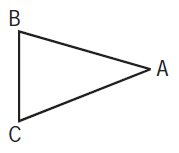Options:

A. 134Pi 1/3 sq. unit

B. 121Pi sq. unit

C. 132Pi sq. unit

D. 176Pi/3 sq. unit

Solution:

CAT Exam Geometry Questions Bank PDF: Ques No 35

A cow is tethered at point A by a rope. Neither the rope nor the cow is allowed to enter ΔABC. If ∠BAC = 30° and I(AB) = I(AC) = 10 m, what is the area that can be grazed by the cow if the length of the rope is 12 m? [CAT 1998]Options:

A. 133Pi 1/6 sq. unit

B. 121Pi sq. unit

C. 132Pi sq. unit

D. 176Pi/3 sq. unit

Solution:

CAT Exam Geometry Questions Bank PDF: Ques No 36

Four identical coins are placed in a square. For each coin the ratio of area to circumference is same as the ratio of circumference to area. Then find the area of the square that is not covered by the coins. [CAT 1998]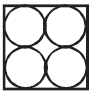Options:

A. 16(π – 1)

B. 16(8 – π)

C. 16(4 – π)

D. 16(4 – π/2)

Solution:

## Geometry Questions for CAT : 1999

CAT Exam Geometry Questions Bank PDF: Ques No 37

Ten points are marked on a straight-line and 11 points are marked on another straight-line. How many triangles can be constructed with vertices from among the above points? [CAT 1999]

Options:

A. 495

B. 550

C. 1045

D. 2475

Solution:

CAT Exam Geometry Questions Bank PDF: Ques No 38

The figure below shows two concentric circles with center O. PQRS is a square inscribed in the outer circle. It also circumscribes the inner circle, touching it at points B, C, D and A. What is the ratio of the perimeter of the outer circle to that of polygon ABCD? [CAT 1999]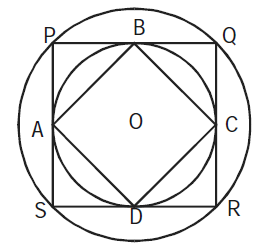Options:

A. Pi/4

B. 3Pi/2

C. Pi/2

D. Pi

Solution:

CAT Exam Geometry Questions Bank PDF: Ques No 39

There is a circle of radius 1 cm. Each member of a sequence of regular polygons S1(n), n = 4, 5, 6, …, where n is the number of sides of the polygon, is circumscribing the circle: and each member of the sequence of regular polygons S2(n), n = 4, 5, 6, … where n is the number of sides of the polygon, is inscribed in the circle. Let L1(n) and L2(n) denote the perimeters of the corresponding polygons of S1(n) and S2(n), then {L1(13) + 2Pi}/L2(17)[CAT 1999]

Options:

A. Greater than Pi/4 and Less than 1

B. Greater than 1 and Less than 2

C. Greater than 2

D. less than Pi/4

Solution:

CAT Exam Geometry Questions Bank PDF: Ques No 40

There A rectangle PRSU, is divided into two smaller rectangles PQTU, and QRST by the line TQ. PQ = 10 cm. QR = 5 cm and RS = 10 cm. Points A, B, F are within rectangle PQTU, and points C, D, E are within the rectangle QRST. The closest pair of points among the pairs (A, C), (A, D), (A, E), (F, C), (F, D), (F, E), (B, C), (B, D), (B, E) are 10Root(3) cm apart.

Which of the following statements is necessarily true? [CAT 1999]

Options:

A. The closest pair of points among the six given points cannot be (F, C).

B. Distance between A and B is greater than that between F and C.

C. The closest pair of points among the six given points is (C, D), (D, E), or (C, E).

D. None of the above

Solution:

CAT Exam Geometry Questions Bank PDF: Ques No 41

A rectangle PRSU, is divided into two smaller rectangles PQTU, and QRST by the line TQ. PQ = 10 cm. QR = 5 cm and RS = 10 cm. Points A, B, F are within rectangle PQTU, and points C, D, E are within the rectangle QRST. The closest pair of points among the pairs (A, C), (A, D), (A, E), (F, C), (F, D), (F, E), (B, C), (B, D), (B, E) are 10Root(3) cm apart.

AB > AF > BF ; CD > DE >CE ; and BF = 6 5 cm. Which is the closest pair of points among all the
six given points?
[CAT 1999]

Options:

A. B, F

B. C, D

C. A, B

D. None of the above

Solution:

## Geometry Questions for CAT : 2000

CAT Exam Geometry Questions Bank PDF: Ques No 42

Consider a circle with unit radius. There are seven adjacent sectors, S1, S2, S3, …, S7, in the circle such that their total area is 1/8 of the area of the circle. Further, the area of the jth sector is twice that of the (j – 1)th sector, for j = 2, …, 7. What is the angle, in radians, subtended by the arc of S1 at the centre of the circle? [CAT 2000]

Options:

A. Pi/508

B. Pi/2040

C. Pi/1016

D. Pi/1524

Solution:

CAT Exam Geometry Questions Bank PDF: Ques No 43

If a, b and c are the sides of a triangle, and a2 + b2 + c2 = bc + ca + ab, then the triangle is [CAT 2000]

Options:

A. Equilateral

B. Isosceles

C. Right Angled

D. Obtuse Angled

Solution:

CAT Exam Geometry Questions Bank PDF: Ques No 44

In the figure, AB = BC = CD = DE = EF = FG = GA. Then ∠DAE is approximately [CAT 2000]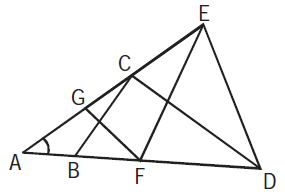Options:

A. 15 Degree

B. 20 Degree

C. 30 Degree

D. 25 Degree

Solution:

CAT Exam Geometry Questions Bank PDF: Ques No 45

ABCDEFGH is a regular octagon. A and E are opposite vertices of the octagon. A frog starts jumping from vertex to vertex, beginning from A. From any vertex of the octagon except E, it may jump to either of the two adjacent vertices. When it reaches E, the frog stops and stays there. Let an be the number of distinct paths of exactly n jumps ending in E. Then what is the value of a2n – 1? [CAT 2000]

Options:

A. 0

B. 4

C. 2n – 1

D. Cannot be determined

Solution:

## Geometry Questions for CAT : 2001

CAT Exam Geometry Questions Bank PDF: Ques No 46

A square, whose side is 2 m, has its corners cut away so as to form an octagon with all sides equal. Then the length of each side of the octagon, in metres, is [CAT 2001]

Options:

A. Root(2)/[Root(2) + 1]

B. 2/[Root(2) + 1]

C. 2/[Root(2) – 1]

D. Root(2)/[Root(2) – 1]

Solution:

CAT Exam Geometry Questions Bank PDF: Ques No 47

A certain city has a circular wall around it, and this wall has four gates pointing north, south, east and west. A house stands outside the city, 3 km north of the north gate, and it can just be seen from a point 9 km east of the south gate. What is the diameter of the wall that surrounds the city? [CAT 2001]

Options:

A. 6 km

B. 9 km

C. 12 km

D. None of these

Solution:

CAT Exam Geometry Questions Bank PDF: Ques No 48

In the diagram, ABCD is a rectangle with AE = EF = FB. What is the ratio of the areas of ΔCEF and that of the rectangle? [CAT 2001]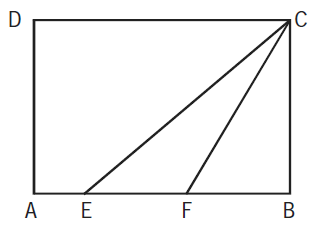Options:

A. 1/6

B. 1/8

C. 1/9

D. None of these

Solution:

CAT Exam Geometry Questions Bank PDF: Ques No 49

A ladder leans against a vertical wall. The top of the ladder is 8 m above the ground. When the bottom of the ladder is moved 2 m farther away from the wall, the top of the ladder rests against the foot of the wall. What is the length of the ladder?  [CAT 2001]

Options:

A. 10

B. 15

C. 20

D. 17

Solution:

CAT Exam Geometry Questions Bank PDF: Ques No 50

Two sides of a plot measure 32 m and 24 m and the angle between them is a perfect right angle. The other two sides measure 25 m each and the other three angles are not right angles. What is the area of the plot? [CAT 2001]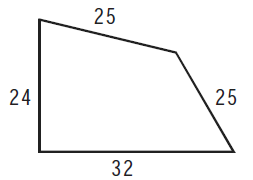Options:

A. 768 m2

B. 534 m2

C. 696.5 m2

D. 684 m2

Solution:

CAT Exam Geometry Questions Bank PDF: Ques No 51

Euclid has a triangle in mind. Its longest side has length 20 and another of its sides has length 10. Its area is 80. What is the exact length of its third side? [CAT 2001]

Options:

A. Root(260)

B. Root(250)

C. Root(240)

D. Root(270)

Solution:

CAT Exam Geometry Questions Bank PDF: Ques No 52

In triangle DEF shown below, points A, B and C are taken on DE, DF and EF respectively such that EC = AC and CF = BC. If ∠D = 400 , then ∠ACB = [CAT 2001]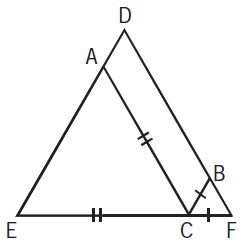Options:

A. 140

B. 70

C. 100

D. None of these

Solution:

CAT Exam Geometry Questions Bank PDF: Ques No 53

Based on the figure below, what is the value of x, if y = 10? [CAT 2001]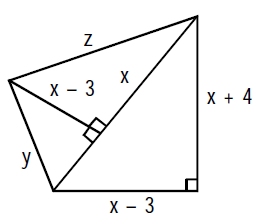Options:

A. 10

B. 11

C. 12

D. None of these

Solution:

CAT Exam Geometry Questions Bank PDF: Ques No 54

A rectangular pool of 20 m wide and 60 m long is surrounded by a walkway of uniform width. If the total area of the walkway is 516 m2, how wide, in metres, is the walkway? [CAT 2001]

Options:

A. 4.3 m

B. 3 m

C. 3.2 m

D. 3.5 m

Solution:

## Geometry Questions for CAT : 2002

CAT Exam Geometry Questions Bank PDF: Ques No 55

In ΔABC, the internal bisector of ∠A meets BC at D. If AB = 4, AC = 3 and ∠A = 60° , then the
[CAT 2002]

Options:

A. 2Root(3)

B. 12Root(3)/7

C. 15Root(3)/8

D. 6Root(3)/7

Solution:

CAT Exam Geometry Questions Bank PDF: Ques No 56

The length of the common chord of two circles of radii 15 cm and 20 cm, whose centers are 25 cm apart, is [CAT 2002]

Options:

A. 24

B. 25

C. 15

D. 20

Solution:

CAT Exam Geometry Questions Bank PDF: Ques No 57

In the figure given below, ABCD is a rectangle. The area of the isosceles right triangle ABE = 7 cm2 ; EC = 3(BE). The area of ABCD (in cm2) is [CAT 2002]Options:

A. 21 cm2

B. 28 cm2

C. 42 cm2

D. 56 cm2

Solution:

CAT Exam Geometry Questions Bank PDF: Ques No 58

Instead of walking along two adjacent sides of a rectangular field, a boy took a short cut along the
diagonal and saved a distance equal to half the longer side. Then the ratio of the shorter side to the
longer side is
[CAT 2002]

Options:

A. 1/2

B. 2/3

C. 1/4

D. 3/4

Solution:

CAT Exam Geometry Questions Bank PDF: Ques No 59

In the given figure, ACB is a right-angled triangle. CD is the altitude. Circles are inscribed within the
ΔACD and ΔBCD. P and Q are the centres of the circles. The distance PQ is
[CAT 2002]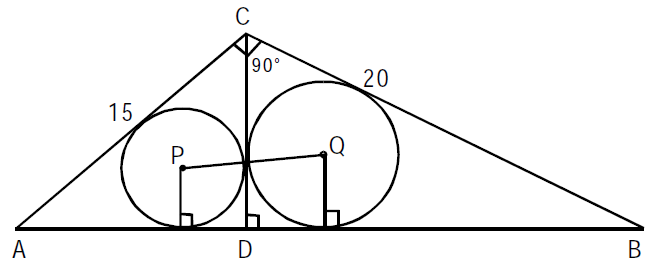Options:

A. 5

B. Root(50)

C. 7

D. 8

Solution:

Direction for Questions 60 and 61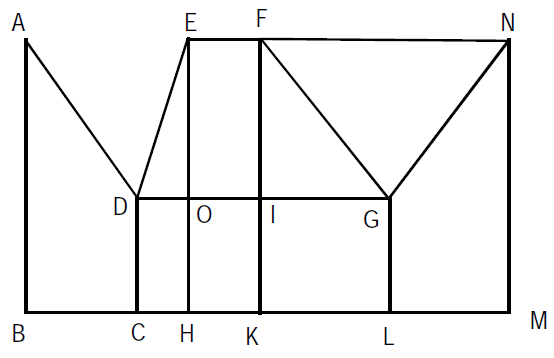In the above diagram, ∠ABC = 90° = ∠DCH = ∠DOE = ∠EHK = ∠FKL = ∠GLM = ∠LMN, AB = BC = 2CH = 2CD = EH = FK = 2HK = 4KL = 2LM = MN

CAT Exam Geometry Questions Bank PDF: Ques No 60

The magnitude of ∠FGO =   [CAT 2002]

Options:

A. 30 Degrees

B. 45 Degrees

C. 60 Degrees

D. None of these

Solution:

CAT Exam Geometry Questions Bank PDF: Ques No 61

What is the ratio of the areas of the two quadrilaterals ABCD to DEFG?   [CAT 2002]

Options:

A. 1 : 2

B. 2 : 1

C. 12 : 7

D. None of these

Solution:

## Geometry Questions for CAT : 2003 Leaked Paper

Direction for Questions 62, 63, 64

In A city has two perfectly circular and concentric ring roads, the outer ring road (OR) being twice as long as the inner ring road (IR). There are also four (straight line) chord roads from E1, the east end point of OR to N2, the north end point of IR; from N1, the north end point of OR to W2, the west end point of IR; from W1, the west end point of OR, to S2, the south end point of IR; and from S1 the south end point of OR to E2, the east end point of IR. Traffic moves at a constant speed of 30π km/hr on the OR road, 20π km/hr on the IR road, and 15Root(5) km/hr on all the chord roads

CAT Exam Geometry Questions Bank PDF: Ques No 62

The ratio of the sum of the lengths of all chord roads to the length of the outer ring road is [CAT 2003 Leaked Paper]

Options:

A. Root(5) : 2

B. Root(5) : 2Pi

C. Root(5) : Pi

D. None of these

Solution:

CAT Exam Geometry Questions Bank PDF: Ques No 63

Amit wants to reach N2 from S1. It would take him 90 minutes if he goes on minor arc S1 – E1 on
OR, and then on the chord road E1 – N2. What is the radius of the outer ring road in kms?
[CAT 2003 Leaked Paper]

Options:

A. 60

B. 40

C. 30

D. 20

Solution:

CAT Exam Geometry Questions Bank PDF: Ques No 64

Amit wants to reach E2 from N1 using first the chord N1 – W2 and then the inner ring road. What
will be his travel time in minutes on the basis of information given in the above question?
[CAT 2003 Leaked Paper]

Options:

A. 60

B. 40

C. 30

D. 20

Solution:

CAT Exam Geometry Questions Bank PDF: Ques No 65

D, E, F are the mid points of the sides AB, BC and CA of triangle ABC respectively. What is the area of DEF in square centimeters?

(A) AD = 1 cm, DF = 1 cm and perimeter of DEF = 3 cm.

(B) Perimeter of ABC = 6 cm, AB = 2 cm, and AC = 2 cm. [CAT 2003 Leaked Paper]

Options:

A. If the question can be answered by one of the statements alone but not by the other.

B. If the question can be answered by using either statement alone.

C. If the question can be answered by using both the statements together, but cannot be answered
by using either statement alone.

D. If the question cannot be answered even by using both the statements together.

Solution:

CAT Exam Geometry Questions Bank PDF: Ques No 66

Each side of a given polygon is parallel to either the X or the Y axis. A corner of such a polygon is said to be convex if the internal angle is 90° or concave if the internal angle is 270°. If the number of convex corners in such a polygon is 25, the number of concave corners must be[CAT 2003 Leaked Paper]

Options:

A. 20

B. 0

C. 21

D. 22

Solution:

CAT Exam Geometry Questions Bank PDF: Ques No 67

There are two concentric circles such that the area of the outer circle is four times the area of the
inner circle. Let A, B and C be three distinct points on the perimeter of the outer circle such that AB
and AC are tangents to the inner circle. If the area of the outer circle is 12 square centimeters then
the area (in square centimeters) of the triangle ABC would be
[CAT 2003 Leaked Paper]

Options:

A. Pi(Root(12))

B. 9/Pi

C. 9Root(3)/Pi

D. 6Root(3)/Pi

Solution:

CAT Exam Geometry Questions Bank PDF: Ques No 68

Three horses are grazing within a semi-circular field. In the diagram given below, AB is the diameter
of the semi-circular field with center at O. Horses are tied up at P, R and S such that PO and RO are
the radii of semi-circles with centers at P and R respectively, and S is the center of the circle touching the two semi-circles with diameters AO and OB. The horses tied at P and R can graze within the respective semi-circles and the horse tied at S can graze within the circle centred at S. The percentage of the area of the semi-circle with diameter AB that cannot be grazed by the horses is nearest to
[CAT 2003 Leaked Paper]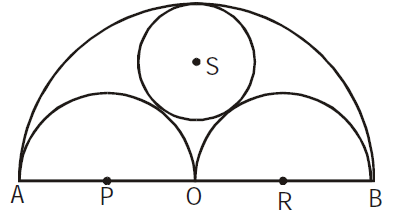Options:

A. 20

B. 28

C. 36

D. 40

Solution:

CAT Exam Geometry Questions Bank PDF: Ques No 69

In the figure below, ABCDEF is a regular hexagon and ∠AOF = 90° . FO is parallel to ED. What is
the ratio of the area of the triangle AOF to that of the hexagon ABCDEF?
[CAT 2003 Leaked Paper]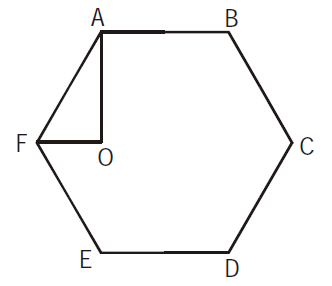Options:

A. 1/12

B. 1/6

C. 1/24

D. 1/18

Solution:

CAT Exam Geometry Questions Bank PDF: Ques No 70

A vertical tower OP stands at the center O of a square ABCD. Let h and b denote the length OP and AB respectively. Suppose ∠APB = 60° then the relationship between h and b can be expressed as [CAT 2003 Leaked Paper]

Options:

A. 2b2 = h2

B. 2h2 = b2

C. 3b2 = 2h2

D. 3h2 = 2b2

Solution:

CAT Exam Geometry Questions Bank PDF: Ques No 71

In the triangle ABC, AB = 6, BC = 8 and AC = 10. A perpendicular dropped from B, meets the side AC at D. A circle of radius BD (with center B) is drawn. If the circle cuts AB and BC at P and Q respectively, the AP:QC is equal to [CAT 2003 Leaked Paper]

Options:

A. 1 : 1

B. 3 : 2

C. 4 : 1

D. 3 : 8

Solution:

CAT Exam Geometry Questions Bank PDF: Ques No 72

In the diagram given below, ∠ABD = ∠CDB = ∠PQD = 90° . If AB:CD = 3:1, the ratio of CD : PQ is [CAT 2003 Leaked Paper]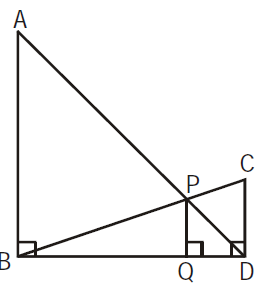Options:

A. 1 : 0.69

B. 1 : 0.75

C. 1 : 0.72

D. None of these

Solution:

CAT Exam Geometry Questions Bank PDF: Ques No 73

There are 8436 steel balls, each with a radius of 1 centimeter, stacked in a pile, with 1 ball on top, 3 balls in the second layer, 6 in the third layer, 10 in the fourth, and so on. The number of horizontal layers in the pile is [CAT 2003 Leaked Paper]

Options:

A. 34

B. 38

C. 36

D. 32

Solution:

CAT Exam Geometry Questions Bank PDF: Ques No 74

In the figure below, AB is the chord of a circle with center O. AB is extended to C such that BC = OB. The straight line CO is produced to meet the circle at D. If ∠ACD = y degrees and ∠AOD = x degrees such that x = ky, then the value of k is [CAT 2003 Leaked Paper]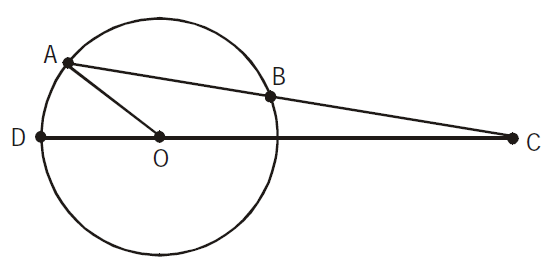Options:

A. 3

B. 2

C. 1

D. None of these

Solution:

CAT Exam Geometry Questions Bank PDF: Ques No 75

In the figure below, the rectangle at the corner measures 10 cm × 20 cm. The corner A of the rectangle is also a point on the circumference of the circle. What is the radius of the circle in cm? [CAT 2003 Leaked Paper]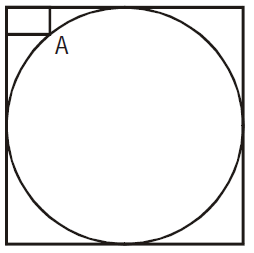Options:

A. 10 cm

B. 40 cm

C. 50 cm

D. None of these

Solution:

## Geometry Questions for CAT : 2003 Retest Paper

Direction for Questions 76, 77, 78

Answer the questions on the basis of the information given below.
Consider three circular parks of equal size with centres at A1, A2, and A3 respectively. The parks touch each other at the edge as shown in the figure (not drawn to scale). There are three paths formed by the triangles A1A2A3, B1B2B3, and C1C2C3,as shown. Three sprinters A, B, and C begin running from points A1, B1 and C1 respectively. Each sprinter traverses her respective triangular path clockwise and returns to her starting point.CAT Exam Geometry Questions Bank PDF: Ques No 76

Let the radius of each circular park be r, and the distances to be traversed by the sprinters A, B and C be a, b and c respectively. Which of the following is true?  [CAT 2003 Retest Paper]

Options:

A. b – a = c – b = 3 Root(3)r

B. b – a = c – b = Root(3)r

C. b = (a + c) / 2 = 2(1 + Root(3)r)

D. c = 2b – a = (2 + Root(3))r

Solution:

CAT Exam Geometry Questions Bank PDF: Ques No 77

Sprinter A traverses distances A1A2, A2A3, and A3A1 at an average speeds of 20, 30 and 15 respectively. B traverses her entire path at a uniform speed of (10Root(3) + 20). C traverses distances C1C2, C2C3 and C3C1 at an average speeds of (40/3)(Root(3) + 1), (40/3)(Root(3) + 1) and 120 respectively. All speeds are in the same unit. Where would B and C be respectively when A finishes her sprint? [CAT 2003 Retest Paper]

Options:

A. B1, C1

B. B3, C3

C. B1, C3

D. B1, Somewhere between C3, C1

Solution:

CAT Exam Geometry Questions Bank PDF: Ques No 78

Sprinters A, B and C traverse their respective paths at uniform speeds of u, v and w respectively. It is known that u2:v2:w2 is equal to Area A: Area B: Area C, where Area A, Area B and Area C are the areas of triangles A1A2A3, B1B2B3, and C1C2C3 respectively. Where would A and C be when B reaches point B3? [CAT 2003 Retest Paper]

Options:

A. A2, C3

B. A3, C3

C. A3, C2

D. Somewhere between A2 and A3, Somewhere between C3 and C1

Solution:

CAT Exam Geometry Questions Bank PDF: Ques No 79

In the figure (not drawn to scale) given below, P is a point on AB such that AP : PB = 4 : 3. PQ is parallel to AC and QD is parallel to CP. In ΔARC, ∠ARC = 90 Degrees , and in ΔPQS , ∠PSQ = 90 Degrees . The length of QS is 6 cm. What is the ratio of AP : PD? [CAT 2003 Retest Paper]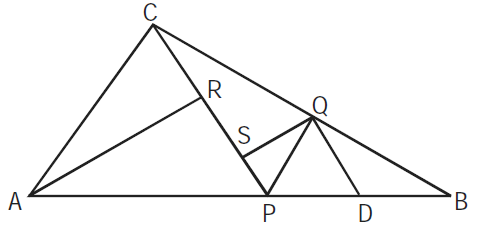Options:

A. 10 : 3

B. 2 : 1

C. 7 : 3

D. 8 : 3

Solution:

CAT Exam Geometry Questions Bank PDF: Ques No 80

In the figure (not drawn to scale) given below, if AD = CD = BC and ∠BCE = 96 Degree , how much is the value of ∠DBC? [CAT 2003 Retest Paper]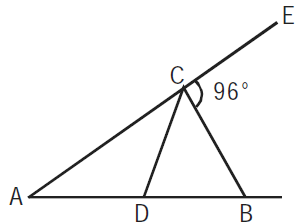Options:

A. 32 Degree

B. 84 Degree

C. 64 Degree

D. Cannot be determined

Solution:

CAT Exam Geometry Questions Bank PDF: Ques No 81

In the figure below (not drawn to scale), rectangle ABCD is inscribed in the circle with centre at O. The length of side AB is greater than side BC. The ratio of the area of the circle to the area of the
rectangle ABCD is π : 3 . The line segment DE intersects AB at E such that ∠ODC = ∠ADE. The ratio AE : AD is
[CAT 2003 Retest Paper]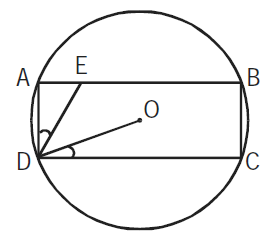Options:

A. 1 : Root(3)

B. 1 : Root(2)

C. 1 : 2Root(3)

D. 1 : 2

Solution:

CAT Exam Geometry Questions Bank PDF: Ques No 82

Let S1 be a square of side a. Another square S2 is formed by joining the mid-points of the sides of S1. The same process is applied to S2 to form yet another square S3, and so on. If A1, A2, A3, … be the areas and P1, P2, P3, … be the perimeters of S1, S2, S3, …, respectively, then the ratio (P1 + P2 + P3 + …)/(A1 + A2 + A3 + …) [CAT 2003 Retest Paper]

Options:

A. 2(1 + Root(2))/a

B. 2(2 – Root(3))/a

C. 2(2 + Root(2))/a

D. 2(2 + 2Root(2))/a

Solution:

CAT Exam Geometry Questions Bank PDF: Ques No 83

In the figure given below (not drawn to scale), A, B and C are three points on a circle with centre O. The chord BA is extended to a point T such that CT becomes a tangent to the circle at point C. If ∠ATC = 30° and ∠ACT = 50°, then the angle ∠BOA is [CAT 2003 Retest Paper]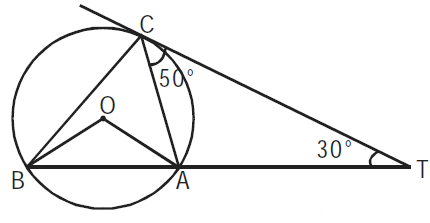Options:

A. 100 Degree

B. 150 Degree

C. 80 Degree

D. Not Possible to Determined

Solution:

CAT Exam Geometry Questions Bank PDF: Ques No 84

Let ABCDEF be a regular hexagon. What is the ratio of the area of the Δ ACE to that of the hexagon ABCDEF? [CAT 2003 Retest Paper]

Options:

A. 1/3

B. 1/2

C. 2/3

D. 5/6

Solution:

CAT Exam Geometry Questions Bank PDF: Ques No 85

A piece of paper is in the shape of a right-angled triangle and is cut along a line that is parallel to the hypotenuse, leaving a smaller triangle. There was 35% reduction in the length of the hypotenuse of the triangle. If the area of the original triangle was 34 square inches before the cut, what is the area (in square inches) of the smaller triangle? [CAT 2003 Retest Paper]

Options:

A. 16.665

B. 16.565

C. 15.465

D. 14.365

Solution:

CAT Exam Geometry Questions Bank PDF: Ques No 86

Two straight roads R1 and R2 diverge from a point A at an angle of 120°. Ram starts walking from point A along R1 at a uniform speed of 3 km/hr. Shyam starts walking at the same time from A along R2 at a uniform speed of 2 km/hr. They continue walking for 4 hr along their respective roads and reach points B and C on R1 and R2 respectively. There is a straight line path connecting B and C. Then Ram returns to point A after walking along the line segments BC and CA. Shyam also returns to A after walking along line segments BC and CA. Their speeds remains unchanged. The time interval (in hours) between Ram’s and Shyam’s return to the point A is [CAT 2003 Retest Paper]

Options:

A. [10Root(19) + 26]/3

B. [2Root(19) + 10]/3

C. [Root(19) + 26]/3

D. [Root(19) + 10]/3

Solution:

## Geometry Questions for CAT : 2004

CAT Exam Geometry Questions Bank PDF: Ques No 87

A rectangular sheet of paper, when halved by folding it at the mid point of its longer side, results in a rectangle, whose longer and shorter sides are in the same proportion as the longer and shorter sides of the original rectangle. If the shorter side of the original rectangle is 2, what is the area of the smaller rectangle [CAT 2004]

Options:

A. 4Root(2)

B. 2Root(2)

C. Root(2)

D. None of these

Solution:

Direction for Questions 88, 89, 90

In the adjoining figure I and II, are circles with P and Q respectively, The two circles touch each other and have common tangent that touches them at points R and S respectively. This common tangent meets the line joining P and Q at O. The diameters of I and II are in the ratio 4 : 3. It is also known that the length of PO is 28 cm.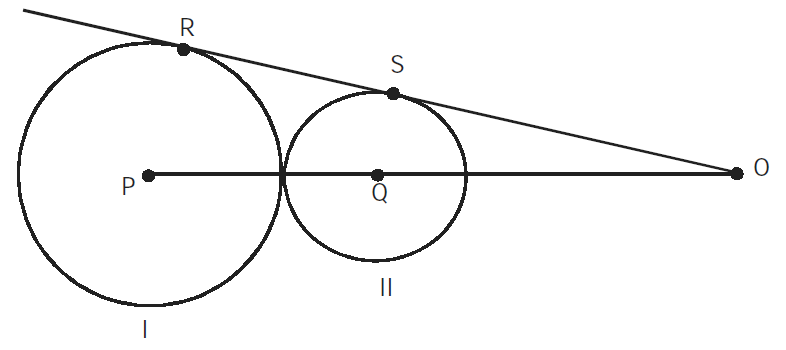CAT Exam Geometry Questions Bank PDF: Ques No 88

What is the ratio of the length of PQ to that of QO? [CAT 2004]

Options:

A. 1 : 4

B. 1 : 3

C. 3 : 8

D. 3 : 4

Solution:

CAT Exam Geometry Questions Bank PDF: Ques No 89

What is the radius of the circle II? [CAT 2004]

Options:

A. 2 cm

B. 3 cm

C. 4 cm

D. 5 cm

Solution:

CAT Exam Geometry Questions Bank PDF: Ques No 90

The length of SO is [CAT 2004]

Options:

A. 2 cm

B. 3 cm

C. 4 cm

D. 5 cm

Solution:

CAT Exam Geometry Questions Bank PDF: Ques No 91

Let C be a circle with centre P0 and AB be a diameter of C. Suppose P1 is the mid point of the line segment P0B, P2 is the mid point of the line segment P1B and so on. Let C1, C2, C3, … be circles with diameters P0P1, P1P2, P2P3… respectively. Suppose the circles C1, C2, C3, … are all shaded. The ratio of the area of the unshaded portion of C to that of the original circle is [CAT 2004]

Options:

A. 8 : 9

B. 9 : 10

C. 10 : 11

D. 11 : 12

Solution:

CAT Exam Geometry Questions Bank PDF: Ques No 92

On a semicircle with diameter AD, chord BC is parallel to the diameter. Further, each of the chords AB and CD has length 2, while AD has length 8. What is the length of BC? [CAT 2004]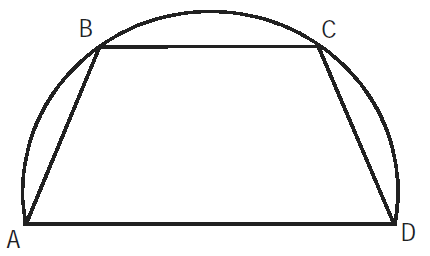Options:

A. 7.5

B. 7

C. 7.75

D. None of these

Solution:

CAT Exam Geometry Questions Bank PDF: Ques No 93

A circle with radius 2 is placed against a right angle. Another smaller circle is also placed as shown in the adjoining figure. What is the radius of the smaller circle? [CAT 2004]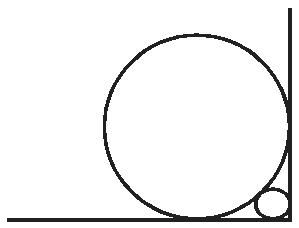Options:

A. 3 – 2Root(2)

B. 4 – 2Root(2)

C. 7 – 4Root(2)

D. 6 – 4Root(2)

Solution:

CAT Exam Geometry Questions Bank PDF: Ques No 94

In the adjoining figure, chord ED is parallel to the diameter AC of the circle. If ∠CBE = 65°, then what is the value of ∠DEC?  [CAT 2004]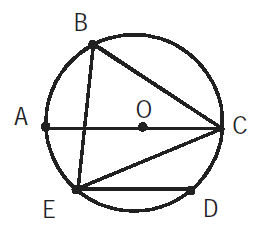Options:

A. 35 Degree

B. 55 Degree

C. 45 Degree

D. 25 Degree

Solution:

## Geometry Questions for CAT : 2005

CAT Exam Geometry Questions Bank PDF: Ques No 95

Two identical circles intersect so that their centers, and the points at which they intersect, form a square of side 1 cm. The area in sq. cm of the portion that is common to the two circles is  [CAT 2005]

Options:

A. Pi/4

B. Pi/2 – 1

C. Pi/5

D. Root(2) – 1

Solution:

CAT Exam Geometry Questions Bank PDF: Ques No 96

What is the distance in cm between two parallel chords of lengths 32 cm and 24 cm in a circle of radius 20 cm?  [CAT 2005]

Options:

A. 1 or 7

B. 2 or 14

C. 3 or 21

D. 4 or 28

Solution:

CAT Exam Geometry Questions Bank PDF: Ques No 97

In the following figure, the diameter of the circle is 3 cm. AB and MN are two diameters such that MN is perpendicular to AB. In addition, CG is perpendicular to AB such that AE : EB = 1:2, and DF is perpendicular to MN such that NL:LM = 1:2. The length of DH in cm is [CAT 2005]Options:

A. 2Root(2) – 1

B. [2Root(2) – 1]/2

C. [3Root(2) – 1]/2

D. [2Root(2) – 1]/3

Solution:

CAT Exam Geometry Questions Bank PDF: Ques No 98

Consider the triangle ABC shown in the following figure where BC = 12 cm, DB = 9 cm, CD = 6 cm and ∠BCD = ∠BAC. What is the ratio of the perimeter of ΔADC to that of the ΔBDC? [CAT 2005]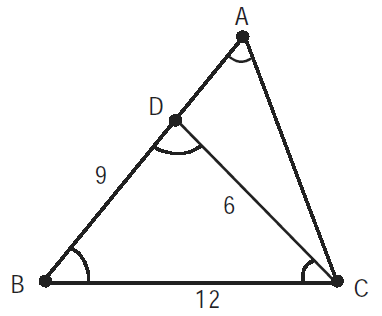Options:

A. 7/9

B. 8/9

C. 6/9

D. 5/9

Solution:

CAT Exam Geometry Questions Bank PDF: Ques No 99

P, Q, S and R are points on the circumference of a circle of radius r, such that PQR is an equilateral
triangle and PS is a diameter of the circle. What is the perimeter of the quadrilateral PQSR?
[CAT 2005]

Options:

A. 2r(1 + Root(3))

B. 2r(2 + Root(3))

C. r(1 + Root(5))

D. 2r + Root(3)

Solution:

## Geometry Questions for CAT : 2006

CAT Exam Geometry Questions Bank PDF: Ques No 100

A semi-circle is drawn with AB as its diameter. From C, a point on AB, a line perpendicular to AB is drawn meeting the circumference of the semi-circle at D. Given that AC = 2 cm and CD = 6 cm, the area of the semi-circle (in sq. cm) will be: [CAT 2006]

Options:

A. 32 π

B. 50 π

C. 40.5 π

D. 81 π

E. Indeterminable

Solution:

Direction for Questions 101 and 102

A punching machine is used to punch a circular hole of diameter two units from a square sheet of aluminium of width 2 units, as shown below. The hole is punched such that the circular hole touches one corner P of the square sheet and the diameter of the hole originating at P is in line with a diagonal of the square.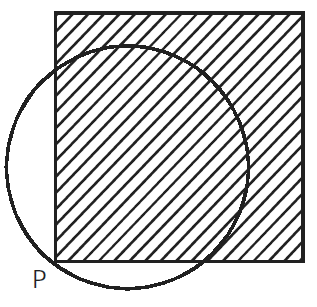CAT Exam Geometry Questions Bank PDF: Ques No 101

The proportion of the sheet area that remains after punching is:  [CAT 2006]

Options:

A. (π + 2)/8

B. (6 – π)/8

C. (4 – π)/4

D. (π  – 2)/4

E. (14 – 3π)/6

Solution:

CAT Exam Geometry Questions Bank PDF: Ques No 102

Find the area of the part of the circle (round punch) falling outside the square sheet.  [CAT 2006]

Options:

A. π/4

B. (π – 1)/2

C. (π – 1)/4

D. (π  – 2)/2

E. (π – 2)/4

Solution:

CAT Exam Geometry Questions Bank PDF: Ques No 103

An equilateral triangle BPC is drawn inside a square ABCD. What is the value of the angle APD in degrees?  [CAT 2006]

Options:

A. 75

B. 90

C. 120

D. 135

E. 150

Solution:

## Geometry Questions for CAT : 2007

CAT Exam Geometry Questions Bank PDF: Ques No 104

Rahim plans to draw a square JKLM with point O on the side JK but is not successful. Why is Rahim unable to draw the square?

A: The length of OM is twice that of OL.

B: The length of OM is 4 cm. [CAT 2007]

Options:

A. The questions can be answered using A alone but not using B alone.

B. The question can be answered using B alone but not using A alone.

C. The question can be answered using A and B together, but not using either A or B alone.

D. The question cannot be answered even using A and B together.

Solution:

CAT Exam Geometry Questions Bank PDF: Ques No 105

Two circles with centers P and Q cut each other at two distinct points A and B. The circles have the
same radii and neither P nor Q falls within the intersection of the circles. What is the smallest range
that includes all possible values of the angle AQP in degrees?
[CAT 2007]

Options:

A. Between 0 and 90

B. Between 0 and 30

C. Between 0 and 60

D. Between 0 and 75

E. Between 0 and 45

Solution:

## Geometry Questions for CAT : 2008

CAT Exam Geometry Questions Bank PDF: Ques No 106

In a triangle ABC, the lengths of the sides AB and AC equal 17.5 cm and 9 cm respectively. Let D be a point on the line segment BC such that AD is perpendicular to BC. If AD = 3 cm, then what is the radius (in cm) of the circle circumscribing the triangle ABC?  [CAT 2008]

Options:

A. 17.05

B. 27.85

C. 22.45

D. 32.25

E. 26.25

Solution:

CAT Exam Geometry Questions Bank PDF: Ques No 107

In a triangle ABC, the lengths of the sides AB and AC equal 17.5 cm and 9 cm respectively. Let D be a point on the line segment BC such that AD is perpendicular to BC. If AD = 3 cm, then what is the radius (in cm) of the circle circumscribing the triangle ABC?  [CAT 2008]

Options:

A. 17.05

B. 27.85

C. 22.45

D. 32.25

E. 26.25

Solution:

CAT Exam Geometry Questions Bank PDF: Ques No 108

Consider obtuse-angled triangles with sides 8 cm, 15 cm and x cm. If x is an integer then how many
such triangles exist?
[CAT 2008]

Options:

A. 5

B. 21

C. 10

D. 15

E. 14

Solution:

CAT Exam Geometry Questions Bank PDF: Ques No 109

Consider a square ABCD with midpoints E, F, G, H of AB, BC, CD and DA respectively. Let L denote the line passing through F and H. Consider points P and Q, on L and inside ABCD such that the angles APD and BQC both equal 120°. What is the ratio of the area of ABQCDP to the remaining area inside ABCD?  [CAT 2008]

Options:

A. 4Root(2)/3

B. 2 + Root(3)

C. [10 – 3Root(3)]/9

D. 1 + 1/Root(3)

E. 2Root(3) – 1

Solution:

CAT Exam Geometry Question Bank PDF: Ques No 110

Two circles, both of radii 1 cm, intersect such that the circumference of each one passes through the centre of the other. What is the area (in sq. cm.) of the intersecting region?  [CAT 2008]

Options:

A. Pi/3 – Root(3)/4

B. 2Pi/3 – Root(3)/2

C. 4Pi/3 – Root(3)/2

D. 4Pi/3 + Root(3)/2

E. 2Pi/3 – Root(3)/2

Solution:

## Geometry Questions for CAT : 2009

CAT Exam Geometry Questions Bank PDF: Ques No 111

M is the center of the circle, Arc(QS) = 10Root(2), Arc(PR) = Arc(RS) and PR || QS. Find the area of shaded region. Use Pi = 3. [CAT 2009]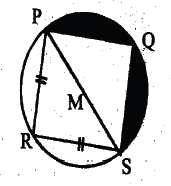Options:

A. 100 sq. unit

B. 114 sq. unit

C. 50 sq. unit

D. 200 sq. unit

Solution:

CAT Exam Geometry Questions Bank PDF: Ques No 112

There are three co-planer parallel lines. If any p points are taken on each of the lines, then find the maximum number of triangles with the vertices of these points   [CAT 2009]

Options:

A. p2(4q – 3)

B. p3(4p – 3)

C. p(4p – 3)

D. p3

Solution:

CAT Exam Geometry Question Bank PDF: Ques No 113

If both ABCD and CDFE are parallelograms. What is (q + r)?

(1) r = 70 degrees,     (2) p = 110 degrees  [CAT 2009]Options:

A. Statement (1) ALONE is sufficient, but statement (2) ALONE is not sufficient.

B. Statement (2) ALONE is sufficient, but statement (1) ALONE is not sufficient.

C. BOTH statement TOGETHER are sufficient, but NEITHER statement ALONE is sufficient.

D. EACH statement ALONE is sufficient.

Solution:

## Geometry Questions for CAT : 2010

CAT Exam Geometry Question Bank PDF: Ques No 114

A pole has to be standing on the boundary of a circular park of diameter 13 meters in such a way that the difference of its distances from two diametrically opposite fixed gates A and B on the boundary is 7 times. The distance of the pole from one of the gates is [CAT 2010]

Options:

A. 8 meters

B. 8.25 meters

C. 5 meters

D. None of these

Solution:

CAT Exam Geometry Question Bank PDF: Ques No 115

From a square piece of card-board measuring 2a on each side of a box with no top is to be formed by cutting out from each corner a square with sides b and bending up the flaps. The value of b for which the box has the greatest volume is [CAT 2010]

Options:

A. b = a/5

B. b = a/4

C. b = 2a/3

D. b = a/2

Solution:

CAT Exam Geometry Question Bank PDF: Ques No 116

The sum of the areas of two circles which touch each other externally is 153Pi. If the sum of their radii is 15, find the ratio of the larger to the smaller radius. [CAT 2010]

Options:

A. 4

B. 2

C. 3

D. None of these

Solution:

CAT Exam Geometry Question Bank PDF: Ques No 117

ABCD is rectangle. The points P and Q lie on AD and AB respectively. If the triangles PAQ, QBC and PCD all have the same areas and BQ = 2, then AQ = [CAT 2010]

Options:

A. 1 + Root(5)

B. 1 – Root(5)

C. Root(7)

D. 2Root(7)

Solution:

## Geometry Questions for CAT : 2011

CAT Exam Geometry Question Bank PDF: Ques No 118

On a plate in the shape of an equilateral triangle ABC with area 16Root(3) sq cm, a rod GD, of height 8 cm, is fixed vertically at the centre of the triangle, G is a point on the plate. If the areas of the triangles AGD and BGD are both equal to 4Root(9) sq cm, find the area of the triangle CGD (in sq cm) [CAT 2011]

Options:

A. 3Root(19)

B. 4Root(19)

C. 12Root(13)

D. None of these

Solution:

CAT Exam Geometry Question Bank PDF: Ques No 119

In the figure alongside, the triangle ABC is an equilateral with area S. M is the mid-point of BC and P is a point on AM extended such that MP = BM. If the semi-circle on AP intersects CB extended at Q and the area of square with MQ as a side is T, which of the following is true? [CAT 2011]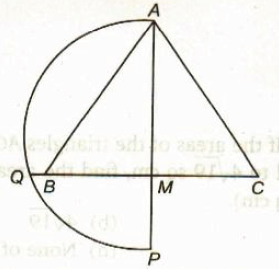Options:

A. T = Root(2)S

B. T = S

C. T = Root(3)S

D. T = 2S

Solution:

CAT Exam Geometry Question Bank PDF: Ques No 120

In the figure alongside, O is the center of the circle and AC the diameter. The line FEG is tangent to the tangent to the circle at E. If angle GEC = 52 degrees, find the value of angle (e + c) [CAT 2011]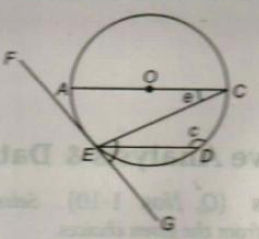Options:

A. 154 Degrees

B. 156 Degrees

C. 166 Degrees

D. 180 Degrees

Solution:

CAT Exam Geometry Question Bank PDF: Ques No 121

Rekha drew a circle of radius 2 cm on a graph paper of grid 1 cm x 1 cm. She then calculated the area of the circle by adding up only the number of full unit-squares that fell within the perimeter of the circle. If the value that Rekha obtained was d sq cm. less than the correct value, then the maximum possible value of d? [CAT 2011]

Options:

A. 6.28

B. 7.28

C. 7.56

D. 8.56

Solution:

## Geometry Questions for CAT : 2012

CAT Exam Geometry Question Bank PDF: Ques No 122

In the figure below, angle MON = angle MPO = angle NQO = 90 Degrees and OQ is the bisector of angle MON and QN = 10, OR = 40/7. Find OP. [CAT 2012]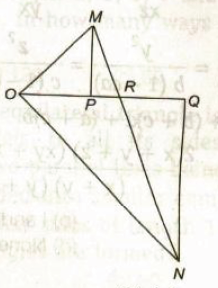Options:

A. 4.8

B. 4.5

C. 4

D. 5

Solution:

CAT Exam Geometry Question Bank PDF: Ques No 123

The side of an equilateral triangle is 10 cm long. by drawing parallels to all its sides, the distance between any two parallel lines being the same. The triangle is divided into smaller equilateral triangle, each of which has sides of length 1 cm. How many such triangles are formed? [CAT 2012]

Options:

A. 60

B. 90

C. 120

D. None of these

Solution:

## Geometry Questions for CAT : 2014

CAT Exam Geometry Question Bank PDF: Ques No 124

If ABCD is a square and BCE is an equilateral triangle, what is the measure of angle∠DEC?  [CAT 2014]

Options:

A. 15 Degrees

B. 30 Degrees

C. 20 Degrees

D. 45 Degrees

Solution:

CAT Exam Geometry Question Bank PDF: Ques No 125

Two circles with centres P and Q cut each other at two distinct points A and B. The circles have the same radii and neither P nor Q falls within the intersection of the circles. What is the smallest range that includes all possible values of the angle AQP in degrees?  [CAT 2014]

Options:

A. Between 0 and 90

B. Between 0 and 30

C. Between 0 and 60

D. Between 0 and 75

Solution:

CAT Exam Geometry Question Bank PDF: Ques No 126

A circle is inscribed in a given square and another circles circumscribed about the square. What is the ratio of the area of the inscribed circle to that of the circumscribed circle?  [CAT 2014]

Options:

A. 2 : 3

B. 3 : 4

C. 1 : 4

D. 1 : 2

Solution:

## Geometry Questions for CAT : 2016

CAT Exam Geometry Question Bank PDF: Ques No 127

In the figure below, P, Q and R are points on a circle with centre O. The tangent to the circle at R intersects secant PQ at T. If the angle QRT = 55 degrees and the angle QTR = 25 degrees, then find the value of angle POQ. [CAT 2016]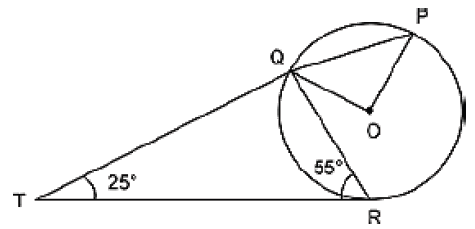Options:

A. 110 Degrees

B. 100 Degrees

C. 90 Degrees

D. 50 Degrees

Solution:

CAT Exam Geometry Question Bank PDF: Ques No 128

In the figure below, BD = 8 cm and DC = 6 cm. AE : ED = 3 : 4. If AF = 12 cm, find AC (in cm) [CAT 2016]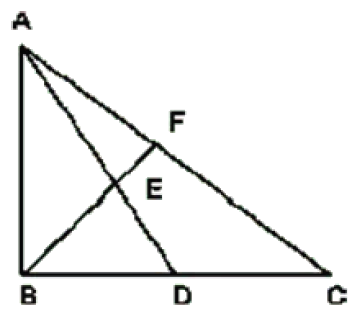Options:

A. 28

B. 38

C. 44

D. 40

Solution:

CAT Exam Geometry Question Bank PDF: Ques No 129

A regular polygon has an even number of sides. If the product of the length of its side and the distance between two opposite sides is 1/4th of its area, find the number of sides it has [CAT 2016]

Options:

A. 6

B. 8

C. 20

D. 16

Solution:

## Geometry Questions for CAT : 2017

CAT Exam Geometry Question Bank PDF: Ques No 130

From a triangle ABC with sides of lengths 40ft, 25 ft and 35ft, a triangular portion GBC is cut off where G is the centroid of triangle ABC. The area in sq ft. of the remaining portion of the triangle ABC is [CAT 2017]

Options:

A. 225Root(3)

B. 500/Root(3)

C. 275/Root(3)

D. 250/Root(3)

Solution:

CAT Exam Geometry Question Bank PDF: Ques No 131

Let ABC be a right angled isosceles triangle with hypotenuse BC. Let BCQ be a semi-circle, away from A, with diameter BC. Let BPC be an arc of a circle centered at A and lying between BC and BQC. If AB has length 6 cm then the area, in sq cm, of the region enclosed by BPC and BQC is [CAT 2017]

Options:

A. 9Pi – 18

B. 18

C. 9Pi

D. 9

Solution:

CAT Exam Geometry Question Bank PDF: Ques No 132

Let ABC be a right angled triangle with BC as the hypotenuse. Length of AB and AC are 15 km and 20 km respectively. The minimum possible time, in minutes, required to reach the hypotenuse from A at a speed of 30 km per hour is [CAT 2017]

Options:

A.

B.

C.

D.

Solution:

CAT Exam Geometry Question Bank PDF: Ques No 133

Let AB, CD, EF, GH and JK be five diameters of a circle with centre at O. in how many ways can three points be chosen out of A, B, C, D, E, F, G, H, J, K and O so as to form a triangle? [CAT 2017]

Options:

A.

B.

C.

D.

Solution:

CAT Exam Geometry Question Bank PDF: Ques No 134

Let ABCDEF be a regular hexagon with each side of length 1 cm. The area (in sq. cm) of a square with AC as one side is  [CAT 2017]

Options:

A. 3Root(2)

B. 3

C. 4

D. Root(3)

Solution:

CAT Exam Geometry Question Bank PDF: Ques No 135

The base of a vertical pillar with uniform cross section is a trapezium whose parallel sides are of lengths 10 cm and 20 cm while the other two sides are of equal length. The perpendicular distance between the parallel sides of the trapezium is 12 cm. If the height of the pillar is 20 cm, then the total area in sq cm of all six surfaces of the pillar is   [CAT 2017]

Options:

A. 1300

B. 1340

C. 1480

D. 1520

Solution:

CAT Exam Geometry Question Bank PDF: Ques No 136

ABCD is a quadrilateral inscribed in a circle with center O. If the angle COD = 120 degrees and the angle BAC = 30 degrees, then the value of angle BCD in degree is is   [CAT 2017]

Options:

A. 90

B. 40

C. 60

D. 50

Solution:

CAT Exam Geometry Question Bank PDF: Ques No 137

If three sides of a rectangular park have a total length 400 ft, then the area of the park is maximum when the length (in ft) of its longer side is [CAT 2017]

Options:

A. 200

B. 120

C. 160

D. 250

Solution:

CAT Exam Geometry Question Bank PDF: Ques No 138

Let P be an interior point of right angled isosceles triangle ABC with hypotenuse AB. If the perpendicular distance of P from each of AB, BC and CA is 4[Root(2) – 1] cm, then the area in sq cm of the triangle ABC is [CAT 2017]

Options:

A. 20

B. 12

C. 16

D. 25

Solution:

## Geometry Questions for CAT : 2018

CAT Exam Geometry Question Bank PDF: Ques No 139

In a circle, two parallel chords on the same side of a diameter have lengths 4 cm and 6 cm. If the distance between these chords is 1 cm, then the radius of the circle, in cm, is [CAT 2018]

Options:

A. Root(13)

B. Root(11)

C. Root(12)

D. Root(14)

Solution:

CAT Exam Geometry Question Bank PDF: Ques No 140

In a circle with the center O and radius 1 cm, an arc AB makes an angle 60 degrees at O. Let R be the region bounded by the radii OA, OB and the arc AB. If C and D are two points on OA and OB respectively such that OC = OD and the area of the triangle OCD is half of R, then the length of OC, in cm, is [CAT 2018]

Options:

A. [Pi/3Root(3)]^(1/2)

B. [Pi/4]^(1/2)

C. [Pi/4Root(3)]^(1/2)

D. [Pi/6]^(1/2)

Solution:

CAT Exam Geometry Question Bank PDF: Ques No 141

In a parallelogram ABCD of area 72 sq cm, the sides CD and AD have lengths 9 cm and 16 cm respectively. Let P be a point on CD such that AP is perpendicular to CD. Then, the area, in sq cm, of the triangle APD is  [CAT 2018]

Options:

A. 24Root(3)

B. 18Root(3)

C. 32Root(3)

D. 12Root(3)

Solution:

CAT Exam Geometry Question Bank PDF: Ques No 142

Let ABCD be a rectangle inscribed in a circle of radius 13 cm. Which one of the following pairs can represent, in cm, then the possible length of breadth of ABCD?  [CAT 2018]

Options:

A. 24, 10

B. 24, 12

C. 25, 9

D. 25, 10

Solution:

CAT Exam Geometry Question Bank PDF: Ques No 143

Given an equilateral triangle T1 with sides 24 cm, a second triangle T2 is formed by joining the midpoints  of the sides of T1. Then, a third triangle T3 is formed by joining the midpoints of the sides of T2. It this process is forming triangle is continued, then the sum of the areas, in sq cm, of infinitely many such triangles T1, T2, T3, … will be  [CAT 2018]

Options:

A. 192Root(3)

B. 164Root(3)

C. 248Root(3)

D. 188Root(3)

Solution:

CAT Exam Geometry Question Bank PDF: Ques No 144

A chord of length 5 cm subtends an angle of 60 degree at the centre of a circle. The length, in cm, of a chord that subtends an angle of the same circle is  [CAT 2018]

Options:

A. 5Root(3)

B. 6Root(2)

C. 2Pi

D. 8

Solution:

CAT Exam Geometry Question Bank PDF: Ques No 145

A parallelogram ABCD has area 48 sq cm. If the length of CD is 8 cm and that of AD is s cm, then which of the following is necessarily true? [CAT 2018]

Options:

A. s < = 6

B. s > = 6

C. s ! = 6

D. 5 < = s < = 7

Solution:

CAT Exam Geometry Question Bank PDF: Ques No 146

The area of a rectangle and the square of its perimeter are in the ratio 1 : 25. Then the lengths of the shorter and longer sides of the rectangle are in the ratio [CAT 2018]

Options:

A. 1 : 3

B. 1 : 4

C. 3 : 8

D. 2 : 9

Solution:

CAT Exam Geometry Question Bank PDF: Ques No 147

From a rectangle ABCD of area 768 sq cm, a semicircular part with diameter AB and the area 72Pi sq cm is removed. The perimeter of the leftover portion, in cm, is [CAT 2018]

Options:

A. 88 + 12Pi

B. 86 + 8Pi

C. 82 + 24Pi

D. 80 + 16Pi

Solution:

CAT Exam Geometry Question Bank PDF: Ques No 148

On a triangle ABC, a circle with diameter BC is drawn, intersecting AB and AC at points P and Q, respectively. If the lengths of AB, AC and CP are 30 cm, 25 cm and 20 cm respectively, the the length of BQ is  [CAT 2018]

Options:

A. 24

B. 20

C. 22

D. 26

Solution: